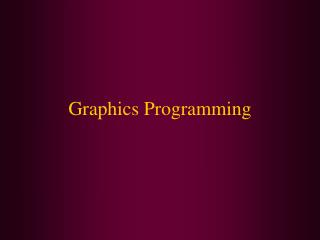# Graphics Programming - PowerPoint PPT PresentationDownload PresentationGraphics Programming

Graphics Programming
Download Presentation## Graphics Programming

- - - - - - - - - - - - - - - - - - - - - - - - - - - E N D - - - - - - - - - - - - - - - - - - - - - - - - - - -
##### Presentation Transcript

1. Graphics Programming

2. In this class we will cover: • Drawing lines, rectangles, and ellipses • Copying an area • Drawing an image

3. Drawing Lines, Rectangles and Ellipses • Java2D oraganizes geometric shapes in OO fashion. There are classes to represent lines, rectangles and ellipses. • Line2D • Rectangle2D • Ellipse2D • These all implement the Shape interface

4. Drawing Shapes • To draw a shape, you first create an object of a class that implements the Shape interface and then call the draw method of the Graphics2D class. Rectangle 2D rect = . . .;g2.draw(rect); • There are two versions of each Shape class • one with float coordinates (conserves memory) • one with double coordinates (easier to use) • Example:Rectangle2D rect = new Rectangle2D.Double(10.0, 25.0, 22.5, 20.0);

5. Drawing Rectangles • Rectangles are simple to construct • Requires four arguments • x- and y-coordinates of the upper-left corner • the width and the height • The Rectangle2D class has over 20 useful methods including: • get Width • getHeight • getCenterX • getCenterY

6. Drawing Rectangles • Sometimes you don’t have the top-left corner readily available. • It is possible to have two diagonal corners of a rectangle that are not the top-left corner and the bottom-right corner. • To create a rectangle in this case use the setFrameFromDiagonal method.Rectangle2D rect = new Rectangle2D.Double();rect.setFrameFromDiagonal(px, py, qx, qy); • If you have a Point2D object, you can also create a rectangle by callingRectangle2D rect = new Rectangle2D.Double(p1, p2);

7. Drawing Ellipses • The class Ellipse2D is inherited from the same Rectangle class that Rectangle2D is. • because of the bounding box surrounding the ellipse • So, creating an Ellipse2D object is very similar to creating a Rectangle2D object.Ellipse2D e = new Ellipse2D.Double(px, py, qx, qy)where px, py are the x- and y-coordinates of the top-left cornerand qx, qy are the x- and y-coordinates of the bottom-right cornerof the bounding box of the ellipse

8. Drawing Lines • To construct a line, simple use the Line2D class. • It too, requires 4 arguments (the x and y coordinates of the start and end positions) • These coordinates can be 2 Point2D objects or 2 pairs of numbers.Line2D line = new Line2D.Double(start, end) orLine2D line = new Line2D.Double(px, py, qx, qy)

9. Filling Shapes • You can fill the interior of closed shape objects with a color. • Simply call the fill instead of draw method on the Graphics object.Rectangle2D rect = new Rectangle2D.Double(x, y, x2, y2);g2.setPaint(Color.red);g2.fill(rect);

10. Images • You can display images on the Graphics object. • You can use images stored locally or someplace on the Internet. • If an image is stored locally call:String filename = “…”;Image image = ImageIO.read(new File(filename)); • If an image is on the Internet, use the url:String filename = “…”;Image image = ImageIO.read(new URL(url); • To draw the image:g.drawImage(image, x, y, null)

11. Copying an Area • copyArea() method - Use to copy an area • Requires 6 parameters • x-coordinate and y-coordinate of the upper-left corner of the area to be copied • Width and height of the area to be copied • The horizontal and vertical displacement of the destination of the copy

12. More on Graphics • We have just begun to touch on the various graphics shapes available. You can also draw: • arcs • polygons • three-dimensional shpes • Java 2D also lets you create higher-quality two-dimensional graphics, images, and text using: • fill patterns such as gradients • strokes that define the width and style of a drawing stroke • anti-aliasing, a graphics technique for producing smoother on-screen graphics • For more info see: http://java.sun.com/docs/books/tutorial/2d/display/strokeandfill.html

13. Example • So, let’s put all of this together in an example: • See:http://www2.bc.edu/~bernier/MC697/LectureNotes/DrawShapesTest.java